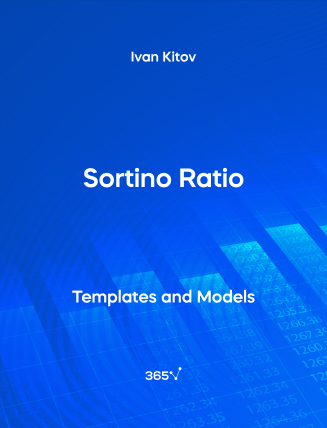## Sortino Ratio – Excel Template###### Ivan Kitov

The Sortino ratio measures the portfolio’s risk-adjusted return. Rational investors use it to maximize their rate of return and minimize the risk of their investment. It is a tool for them to compare different securities. The Sortino ratio is similar to the Sharpe ratio but instead of the overall standard deviation, the metric uses the downside deviation, and not the overall standard deviation of the portfolio’s returns. In the nominator, you have the portfolio’s excess return where you subtract its return from a risk-free rate. And in the denominator, you have the standard deviation of negative returns.

This open-access Excel template is a useful tool for statisticians, financial analysts, data analysts, and portfolio managers.

Sortino Ratio is among the topics included in the Portfolio Management module of the CFA Level 1 Curriculum. Gain valuable insights into the subject with our Portfolio Management course.

You can also explore other related templates such as—Information Ratio, Sharpe Ratio, and Treynor Ratio.Top